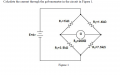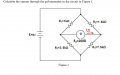# wheatstone bridg...how found ig..??

#### astastg

Joined Nov 28, 2015
3
helllo all..

this my first time here..so welcom all

amm..yeah here we go!..

guys..how i can found ig from this form ..

and what does mean by prove my rsult in any simulation software,..?

any one can hellp me to draw it..?can we put ig over there..?Last edited:

#### Dodgydave

Joined Jun 22, 2012
11,147
Work out the voltage on each side of the bridge using ohms law, then subtract one side from the other to give the voltage across the meter, and that will give you its current and direction.

Ans 1.49mA right to left

Last edited:

#### astastg

Joined Nov 28, 2015
3
Work out the voltage on each side of the bridge using ohms law, then subtract one side from the othe to give the voltage across the meter, and that will give you its current and direction.

oh ..yeah i see i will do this steps thanx
but did you know which best program can use for draw that circuit..

Q...:
and what does mean by prove my rsult in any simulation software,.

#### Dodgydave

Joined Jun 22, 2012
11,147
To prove,means; to make sure it works, you have got the correct answer.

#### RRITESH KAKKAR

Joined Jun 29, 2010
2,829

#### WBahn

Joined Mar 31, 2012
29,489
Work out the voltage on each side of the bridge using ohms law, then subtract one side from the other to give the voltage across the meter, and that will give you its current and direction.
Except that since the bridge isn't balanced you can't use Ohm's Law to determine the voltage on each side of it.

There are several ways to tackle the problem, depending on how much analysis background you have at this point. In the order that you likely are exposed to them, you have brute force KVL/KCL, nodal analysis, mesh analysis, delta-wye transforms, and Thevenin equivalent circuits.

What methods do you know so far?

As for "prove your result in any simulation software", a better phrasing would be to verify your result, but what they are asking you do to is simulate the circuit, apparently using any simulation software of your choosing, and show that the simulator yields the same results that your calculations do. A lot of people here use LTSpice, which is free and fairly easy to use.

#### WBahn

Joined Mar 31, 2012
29,489
First, this is homework, so just offering up answers doesn't help the TS learn.

Second, ask if your answer makes sense. Look at the original circuit and determine whether ig, as the TS defined it, will be positive or negative?

Next, put some bounds on what the answer can be by determining the voltage across the galvanometer assuming that it has infinite resistance. The absolute most current that could possibly flow in the galvanometer would be this voltage divided by the actual galvanometer resistance.

•RRITESH KAKKAR

#### astastg

Joined Nov 28, 2015
3
First, this is homework, so just offering up answers doesn't help the TS learn.

Second, ask if your answer makes sense. Look at the original circuit and determine whether ig, as the TS defined it, will be positive or negative?

Next, put some bounds on what the answer can be by determining the voltage across the galvanometer assuming that it has infinite resistance. The absolute most current that could possibly flow in the galvanometer would be this voltage divided by the actual galvanometer resistance.

yeah on the way..^^
thanx Dr.WBahn

#### RRITESH KAKKAR

Joined Jun 29, 2010
2,829
First, this is homework, so just offering up answers doesn't help the TS learn.

Second, ask if your answer makes sense. Look at the original circuit and determine whether ig, as the TS defined it, will be positive or negative?

Next, put some bounds on what the answer can be by determining the voltage across the galvanometer assuming that it has infinite resistance. The absolute most current that could possibly flow in the galvanometer would be this voltage divided by the actual galvanometer resistance.
Just guessed i am not sure

#### WBahn

Joined Mar 31, 2012
29,489
Just guessed i am not sure
Please don't just throw out guesses in someone else's homework thread. People start such threads because they are struggling with some aspect of it and random guesses not only don't help, but they are likely to confuse the TS.

#### RRITESH KAKKAR

Joined Jun 29, 2010
2,829

#### WBahn

Joined Mar 31, 2012
29,489
Can you teach me?
Previous experience indicates that the chance for success is very low.

•RRITESH KAKKAR

#### RRITESH KAKKAR

Joined Jun 29, 2010
2,829